﻿ 农村人居环境满意度研究——以日照市巨峰镇为例 Study on Satisfaction of Rural Habitat Environment—Taking Jufeng Town of Rizhao City as an Example

Geographical Science Research
Vol. 08  No. 02 ( 2019 ), Article ID: 30035 , 12 pages
10.12677/GSER.2019.82016

Study on Satisfaction of Rural Habitat Environment

—Taking Jufeng Town of Rizhao City as an Example

Yuanhui Liu, Hui Wang*

School of Geography and Tourism, Qufu Normal University, Rizhao Shandong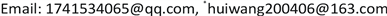Received: Apr. 8th, 2019; accepted: Apr. 23rd, 2019; published: Apr. 30th, 2019ABSTRACT

The quality of rural human settlements is closely related to the living, production and ecological environment of rural residents. Improving rural human settlements is an important part of the strategy of China’s Rural Revitalization. From the perspective of rural residents, this study selected 26 specific indicators from five aspects: natural ecological environment, residential environment, infrastructure, public service facilities and social and human environment to construct the evaluation index system of rural human settlements environmental satisfaction. On the basis of interviews with villages and household surveys, the fuzzy comprehensive evaluation method was used to evaluate the satisfaction of the rural human settlements of 23 villages in Jufeng Town, Rizhao City. On this basis, the model is derived by factor importance, and the four-quadrant maps are used to find out the key factors for improving the rural living environment. The research shows that the overall level of rural human settlements satisfaction is high, there are internal differences; and the villagers have inconsistent evaluation of the importance and satisfaction of rural human settlements, drinking water quality, air quality, building density, heating facilities, educational facilities and medical facilities are characterized by high importance-low satisfaction, and should be given full attention in the future rectification of rural human settlements.

Keywords:Rural Settlement, Satisfaction Degree, Fuzzy Comprehensive Evaluation, Satisfaction Index Model, Suggestions

——以日照市巨峰镇为例1. 引言

2. 研究区与数据获取

2.1. 研究区域概况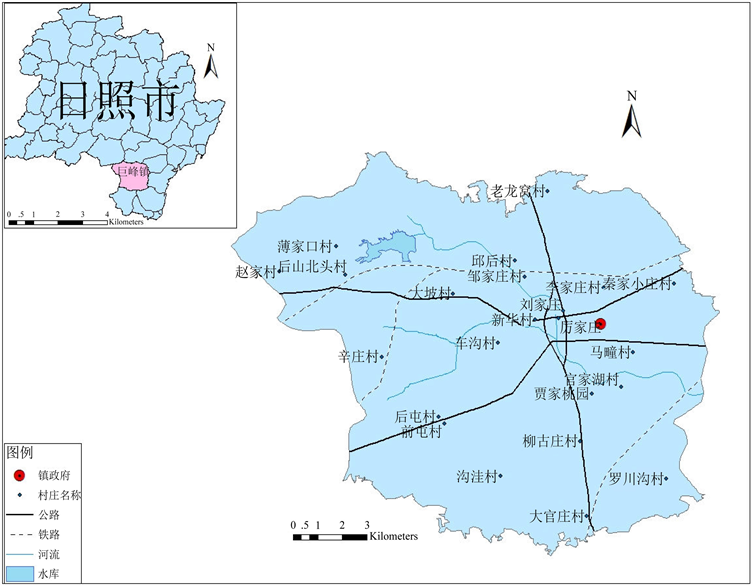Figure 1. Schematic diagram of the location of the village in the study area

2.2. 数据获取Table 1. Basic information of the sample of Jufeng Town

3. 方法选择与模型构建

3.1. 方法选择

3.2. 指标体系构建Table 2. Rural human settlements environmental satisfaction evaluation index system and weight

3.3. 计算过程

1) 建立评价子集和等级标准集

$U=\left(\begin{array}{c}{u}_{1j}\\ {u}_{2j}\\ ⋮\\ {u}_{nj}\end{array}\right)=\left(\begin{array}{cccc}{u}_{11}& {u}_{12}& \cdots & {u}_{1m}\\ {u}_{21}& {u}_{22}& \cdots & {u}_{2m}\\ ⋮& ⋮& \ddots & ⋮\\ {u}_{n1}& {u}_{n2}& \cdots & {u}_{nm}\end{array}\right)$ (3-1)

V = (V1, V2, V3, V4, V5) = (非常不满意，不满意，一般，满意，非常满意)

2) 构建模糊关系矩阵

${R}_{i}=\left(\begin{array}{cccc}{r}_{11}& {r}_{12}& \cdots & {r}_{1n}\\ {r}_{21}& {r}_{22}& \cdots & {r}_{2n}\\ \cdots & \cdots & \cdots & \cdots \\ {r}_{m1}& {r}_{m2}& \cdots & {r}_{mn}\end{array}\right)$ (3-2)

3) 建立一级模糊评价

$W\circ R=\left({w}_{1},{w}_{2},\cdots ,{w}_{m}\right)\circ \left(\begin{array}{cccc}{r}_{11}& {r}_{12}& \cdots & {r}_{1n}\\ {r}_{21}& {r}_{22}& \cdots & {r}_{2n}\\ \cdots & \cdots & \cdots & \cdots \\ {r}_{m1}& {r}_{m2}& \cdots & {r}_{mn}\end{array}\right)=\left({b}_{1},{b}_{2},\cdots ,{b}_{n}\right)=B$ (3-3)

4) 将一级模糊评价的结果作为二级模糊评价的关系矩阵。利用一级模糊评价的方法，进而得到农村人居环境满意度的二级模糊综合评价结果。

5) 村民满意度的综合评价得分

$C=\frac{\underset{j=1}{\overset{n}{\sum }}{b}_{j}^{t}{v}_{k}}{\underset{j=1}{\overset{n}{\sum }}{b}_{j}^{t}}$ (3-4)

4. 评价结果与分析

4.1. 总体满意度分析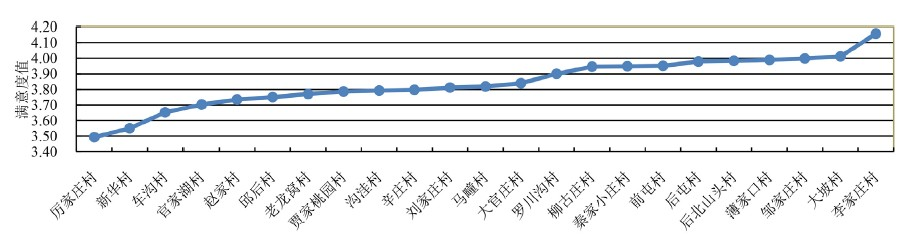Figure 2. Overall satisfaction map of the village human settlements

4.2. 子系统满意度分析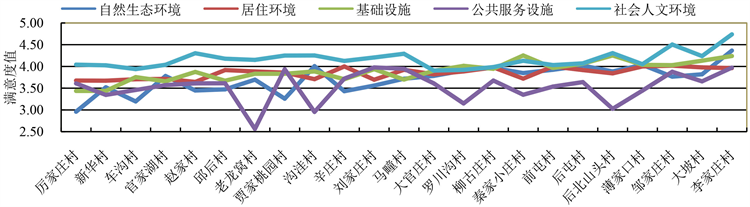Figure 3. Substitute satisfaction graph of the village human settlements

4.3. 各要素满意度分析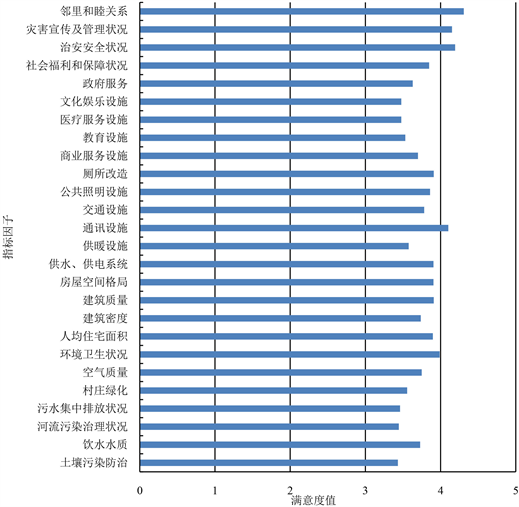Figure 4. Satisfaction evaluation value map of each factor indicator factor

4.4. 满意度重要性分析

4.4.1. 过程分析

${A}_{i}=\underset{j=1}{\overset{j}{\sum }}{X}_{j}{Y}_{ij}\left(i=1,2,\cdots ,26;j=1,2,3,4,5\right)$ (4-1)Table 3. Table of importance and satisfaction of each specific indicator

4.4.2. 结果分析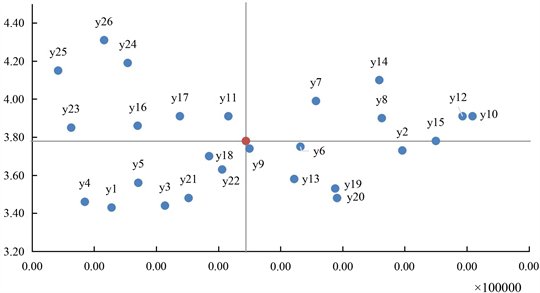Figure 5. Classification of importance and satisfaction of each indicator

5. 结论与建议

5.1. 研究结论

1) 农村人居环境满意度较高

2) 亟待改善的重点依然存在

5.2. 对策启示

1) 完善生态文明建设，精准改善生态环境

2) 加大基础设施投入，提升服务质量

Study on Satisfaction of Rural Habitat Environment—Taking Jufeng Town of Rizhao City as an Example[J]. 地理科学研究, 2019, 08(02): 149-160. https://doi.org/10.12677/GSER.2019.82016

1. 1. 吴良镛. 人居环境科学导论[M]. 北京: 中国建筑工业出版社, 2001: 4-36.

2. 2. 莫妮娜. 从环境行为学探讨新农村人居环境与乡土景观的协调发展[J]. 软科学, 2009, 25(5): 84-88.

3. 3. 李昌浩, 朱晓东, 李杨帆, 等. 快速城市化地区农村集中住宅区和生态人居环境建设研究[J]. 重庆建筑大学学报, 2007, 29(5): 1-5.

4. 4. 蒋淑玲, 王宏波. 新农村建设中农村人居环境建设存在的问题及其对策思考: 以湖南省衡阳市为例[J]. 农业经济, 2010(5): 30-32.

5. 5. 晋培育, 李雪铭, 冯凯. 辽宁城市人居环境竞争力的时空演变与综合评价[J]. 经济地理, 2011, 31(10): 1638-1644.

6. 6. 封志明, 唐焰, 杨艳昭, 等. 基于GIS的中国人居环境指数模型建立与应用[J]. 地理学报, 2008, 63(12): 1327-1336.

7. 7. 李华生, 徐瑞祥, 高中贵, 等. 城市尺度人居环境质量评价研究[J]. 人文地理, 2005, 20(1): 1-5.

8. 8. 侯爱敏, 袁中金. 试论城镇人居环境中的生产环境[J]. 人文地理, 2008, 23(2): 73-76.

9. 9. 李雪铭, 李婉娜. 1990年代以来大连城市人居环境与经济协调发展定量分析[J]. 经济地理, 2005, 25(3): 383-386.

10. 10. 李伯华, 刘沛林, 窦银娣. 乡村人居环境系统的自组织演化机理研究[J]. 经济地理, 2014, 34(9): 130-136.

11. 11. 孙慧波, 赵霞. 中国农村人居环境质量评价及差异化治理策略[J]. 西安交通大学学报, 2018, 12(5): 1-15.

12. 12. 郜慧, 金家胜, 李锋, 等. 中国省域农村人居环境建设评价及发展对策[J]. 生态与农村环境学报, 2015, 31(6): 835-843.

13. 13. 李伯华, 刘传明, 曾菊新. 乡村人居环境的居民满意度评价及其优化策略研究——以石首市久合垸乡为例[J]. 人文地理, 2009, 24(1): 28-32.

14. 14. 周侃, 蔺雪芹, 申玉铭, 等. 京郊新农村建设人居环境质量综合评价[J]. 地理科学进展, 2011, 30(3): 261-368.

15. 15. 吴良镛. 芒福德的学术思想及其对人居环境学建设的启示[J]. 城市规划, 1996(1): 30-31.

16. 16. 刘春艳, 李秀霞, 刘雁. 吉林省乡村人居环境满意度评价与优化[J]. 天津师范大学学报(自然科学版), 2012, 32(3): 54-59.

17. 17. 蔡少华. 闵行区村庄改造农民满意度研究[D]: [硕士学位论文]. 上海: 上海交通大学, 2012.

18. 18. 张萌, 郑华伟, 高春雨, 等. 基于农民主体视角的村庄环境整治满意度研究——以江苏省4个地区的调查为例[J]. 中国农业资源与区划, 2018, 39(4): 145-151.

19. 19. 朱媛媛, 甘依霖, 曾菊新, 等. 基于乡村振兴战略的人居文化环境质量演变驱动机制研究——以长江中游城市群为例[J]. 地理科学, 2018, 38(11): 1855-1863.

20. 20. 王慧杰. 基于AHP-模糊综合评价法的流域生态补偿政策评估研究[D]: [硕士学位论文]. 北京: 中国环境科学研究院, 2015: 33-34.

21. 21. 白梅. 基于模糊综合评价法的网络购物顾客满意度测评研究[D]: [硕士学位论文]. 大连: 东北财经大学, 2012.

22. 22. 殷冉. 基于村民意愿的乡村人居环境改善研究[D]: [硕士学位论文]. 南京: 南京师范大学, 2013.

23. NOTES

*通讯作者。